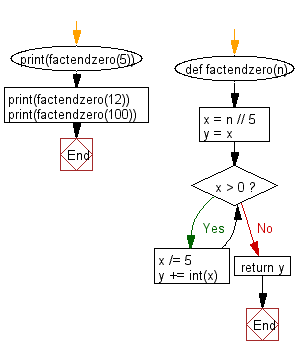﻿ Python: Find the number of zeros at the end of a factorial of a given positive number - w3resource# Python: Find the number of zeros at the end of a factorial of a given positive number

## Python Basic - 1: Exercise-20 with Solution

Write a Python program to find the number of zeros at the end of a factorial of a given positive number.

Range of the number(n): (1 ≤ n ≤ 2*109).

Sample Solution:

Python Code:

``````def factendzero(n):
x = n // 5
y = x
while x > 0:
x /= 5
y += int(x)
return y

print(factendzero(5))
print(factendzero(12))
print(factendzero(100))
``````

Sample Output:

```1
2
24
```

Pictorial Presentation:Flowchart:## Visualize Python code execution:

The following tool visualize what the computer is doing step-by-step as it executes the said program:

Python Code Editor:

Have another way to solve this solution? Contribute your code (and comments) through Disqus.

What is the difficulty level of this exercise?

Test your Programming skills with w3resource's quiz.

﻿

## Python: Tips of the Day

Iterating over dictionaries using 'for' loops:

I am a bit puzzled by the following code: d = {'x': 1, 'y': 2, 'z': 3} for key in d: print key, 'corresponds to', d[key] What I don't understand is the key portion. How does Python recognize ...

key is just a variable name.

```for key in d:
```

For Python 3.x:

```for key, value in d.items():
```

For Python 2.x:

```for key, value in d.iteritems():
```

To test for yourself, change the word key to poop.

In Python 3.x, iteritems() was replaced with simply items(), which returns a set-like view backed by the dict, like iteritems() but even better. This is also available in 2.7 as viewitems().

The operation items() will work for both 2 and 3, but in 2 it will return a list of the dictionary's (key, value) pairs, which will not reflect changes to the dict that happen after the items() call. If you want the 2.x behavior in 3.x, you can call list(d.items()).

Ref: https://bit.ly/37dm0Qo